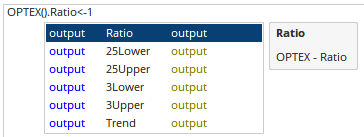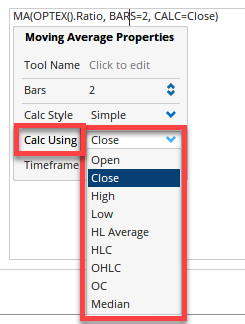Select Page

# Optex Bands

Optuma Forums Optuma Scripting Optex Bands

Viewing 6 posts - 1 through 6 (of 6 total)
• Author
Posts
• #54301

Hi,

I have a question concerning a script for coloring bars according to values of the “Ratio Line” of the Optex Bands indicator.

What I want is a script that colors the bars of e.g. the S&P 500 in green when the “Ratio Line” of the Optex Bands indicator is less than -1.

e.g. Ratio Line Optex Bands<=-1

I know how the settings have do be made in the “Properties” panel of the chart for coloring the bars. I only need the script.

Thomas

#54305

Hi Thomas,

Simply type OPTEX(). and then select the output value required, ie Ratio

OPTEX().Ratio<=-1#54307

Hi Darren,

The script works of course fine.

#54313

Hi,

I want just a bit modify the Optex Bands indicator in that way that I want calculate a moving average of the “Ratio Line”. On the chart this is of course no problem but I have some problems with the script.

I have tried the following but it does not work:

MA(CALC=OPTEX().Ratio, STYLE=Weighted, BARS=2)<=0

The script without a moving average is:

OPTEX().Ratio<=0

I thought I can change the “Close” element of “CALC=Close” with CALC=OPTEX().Ratio but as already mentioned it does not work.

Can anyone give me some advice in this issue?

Thank you very much.

#54317

Hi Thomas,

The CALC field refers to the MA, eg based on closing prices, high, etc. Click on the properties box to see available options:Instead, the item that the moving average is calculating needs to be separated by a comma:

MA(OPTEX().Ratio, BARS=2, CALC=Close, STYLE=Weighted)<=0

For more examples of ‘nesting’ functions see the first lecture here:

https://learn.optuma.com/course/intermediate-scripting/

#54325

Hi Darren,

many thanks again for your help. The assistance I already get from you is priceless!

My next step will be the scripting courses. I have already downloaded all courses and example materials but had not yet the time to work through.

Thomas

Viewing 6 posts - 1 through 6 (of 6 total)
• You must be logged in to reply to this topic.